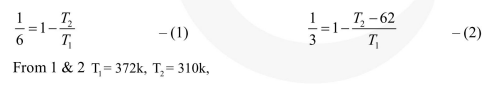# A Carnot engine operating between temperatures`
Question:

A Carnot engine operating between temperatures $T_{1}$ and $T_{2}$ has efficientcy $\frac{1}{6}$. When $T_{2}$ is lowered

by $62 \mathrm{~K}$, its efficiency increases to $\frac{1}{3}$. Then $\mathrm{T}_{1}$ and $\mathrm{T}_{2}$ are, respectively:-

1. $330 \mathrm{~K}$ and $268 \mathrm{~K}$

2. $310 \mathrm{~K}$ and $248 \mathrm{~K}$

3. $372 \mathrm{~K}$ and $310 \mathrm{~K}$

4. $372 \mathrm{~K}$ and $330 \mathrm{~K}$

Correct Option: , 3

Solution: